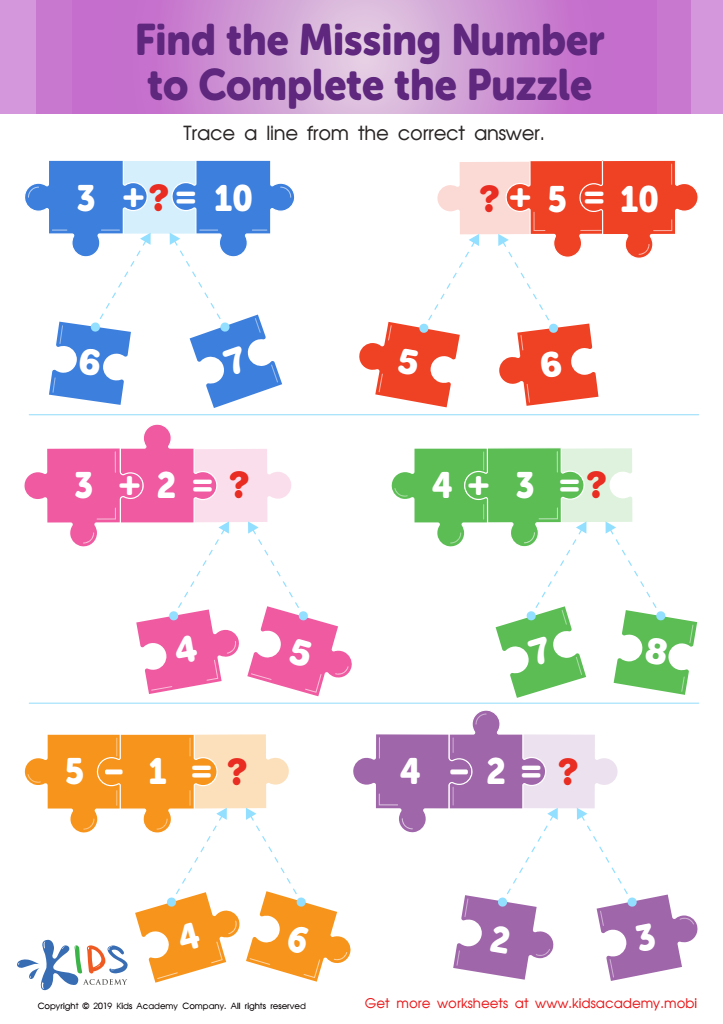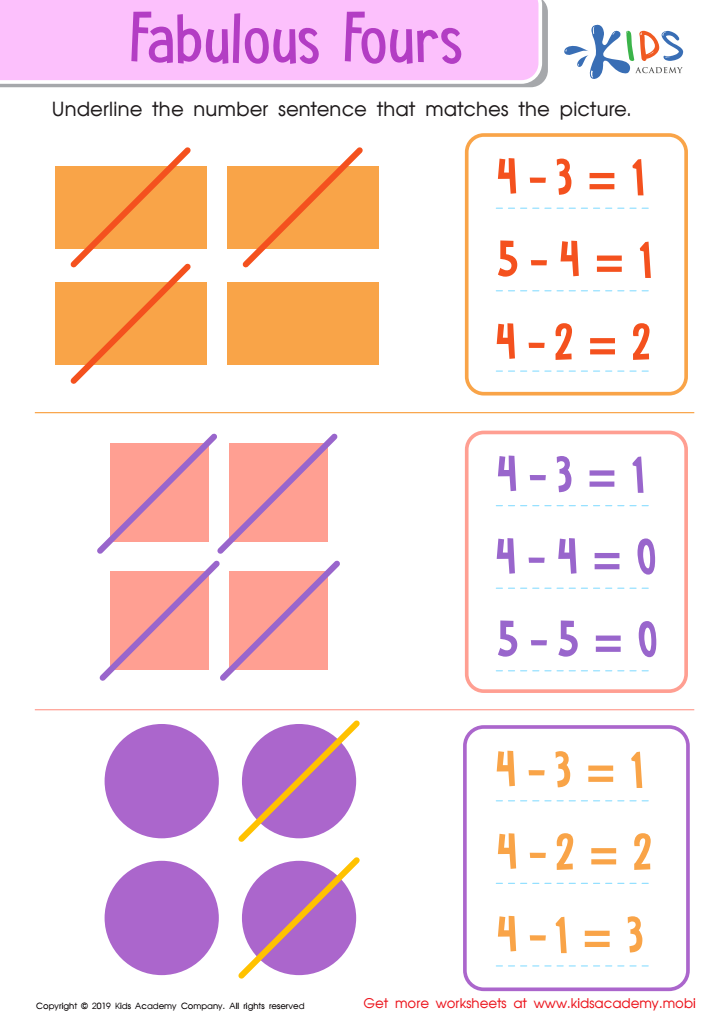# Extra Challenge Online SubtractionWorksheets for Kids

19 filtered results

Clear all filters
Favorites
Online only

19 filtered results

Difficulty Level

Standards
Favorites
Online only
• Online only
• Subtraction
• Extra ChallengeFind the Missing Number to Complete the Puzzle Worksheet
Teach math with fun puzzles! Have kids solve the six equations and trace lines to the correct answer. Puzzles engage minds and help students learn. Try this worksheet to make math learning fun.
Find the Missing Number to Complete the Puzzle Worksheet
Worksheet
Find the Missing Number to Complete the Puzzle Worksheet
Kindergarten
Subtraction
WorksheetFruity Fives! Worksheet
This tracing sheet has three equations for your kids to solve. Make learning fun with colorful objects and text! They may need help, so be prepared to assist them. Help them solve the equations and trace a line to the correct answer.
Fruity Fives! Worksheet
Worksheet
Fruity Fives! Worksheet
In this colorful worksheet, there are three simple equations which your kids must solve. It …
Kindergarten
Subtraction
Worksheet